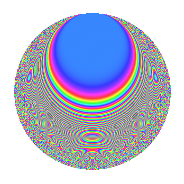# Properties

 Label 1145.2.aLevel 1145 Weight 2 Character orbit a Rep. character $$\chi_{1145}(1,\cdot)$$ Character field $$\Q$$ Dimension 77 Newforms 6 Sturm bound 230 Trace bound 1

# Related objects

## Defining parameters

 Level: $$N$$ = $$1145 = 5 \cdot 229$$ Weight: $$k$$ = $$2$$ Character orbit: $$[\chi]$$ = 1145.a (trivial) Character field: $$\Q$$ Newforms: $$6$$ Sturm bound: $$230$$ Trace bound: $$1$$ Distinguishing $$T_p$$: $$2$$

## Dimensions

The following table gives the dimensions of various subspaces of $$M_{2}(\Gamma_0(1145))$$.

Total New Old
Modular forms 116 77 39
Cusp forms 113 77 36
Eisenstein series 3 0 3

The following table gives the dimensions of the cuspidal new subspaces with specified eigenvalues for the Atkin-Lehner operators and the Fricke involution.

$$5$$$$229$$FrickeDim.
$$+$$$$+$$$$+$$$$16$$
$$+$$$$-$$$$-$$$$22$$
$$-$$$$+$$$$-$$$$22$$
$$-$$$$-$$$$+$$$$17$$
Plus space$$+$$$$33$$
Minus space$$-$$$$44$$

## Trace form

 $$77q - 3q^{2} + 79q^{4} + q^{5} - 4q^{6} + 4q^{7} - 15q^{8} + 73q^{9} + O(q^{10})$$ $$77q - 3q^{2} + 79q^{4} + q^{5} - 4q^{6} + 4q^{7} - 15q^{8} + 73q^{9} + q^{10} - 8q^{11} - 16q^{12} + 10q^{13} - 12q^{14} + 79q^{16} - 6q^{17} - 23q^{18} + 7q^{20} + 28q^{21} - 36q^{22} - 8q^{23} - 20q^{24} + 77q^{25} + 18q^{26} - 12q^{27} + 4q^{28} - 18q^{29} + 8q^{30} + 4q^{31} - 43q^{32} - 12q^{33} - 10q^{34} - 8q^{35} + 51q^{36} + 10q^{37} - 32q^{38} - 40q^{39} + 9q^{40} - 14q^{41} - 12q^{42} + 12q^{43} + 5q^{45} + 36q^{46} - 24q^{47} - 20q^{48} + 101q^{49} - 3q^{50} - 20q^{51} + 6q^{52} + 10q^{53} + 24q^{54} - 4q^{55} - 36q^{56} + 8q^{57} - 6q^{58} - 12q^{59} + 12q^{60} + 14q^{61} + 4q^{62} + 59q^{64} - 14q^{65} + 20q^{66} - 66q^{68} + 24q^{69} + 24q^{70} - 47q^{72} - 2q^{73} - 14q^{74} - 8q^{76} - 16q^{77} - 32q^{78} + 15q^{80} + 61q^{81} - 50q^{82} - 4q^{83} + 104q^{84} + 2q^{85} - 80q^{86} + 12q^{87} - 148q^{88} + 18q^{89} - 23q^{90} + 8q^{91} - 12q^{92} + 40q^{93} - 32q^{94} + 12q^{95} - 52q^{96} + 10q^{97} + 77q^{98} - 36q^{99} + O(q^{100})$$

## Decomposition of $$S_{2}^{\mathrm{new}}(\Gamma_0(1145))$$ into irreducible Hecke orbits

Label Dim. $$A$$ Field CM Traces A-L signs $q$-expansion
$$a_2$$ $$a_3$$ $$a_5$$ $$a_7$$ 5 229
1145.2.a.a $$1$$ $$9.143$$ $$\Q$$ None $$-1$$ $$-2$$ $$-1$$ $$-4$$ $$+$$ $$+$$ $$q-q^{2}-2q^{3}-q^{4}-q^{5}+2q^{6}-4q^{7}+\cdots$$
1145.2.a.b $$2$$ $$9.143$$ $$\Q(\sqrt{2})$$ None $$2$$ $$-2$$ $$-2$$ $$0$$ $$+$$ $$-$$ $$q+q^{2}+(-1+\beta )q^{3}-q^{4}-q^{5}+(-1+\cdots)q^{6}+\cdots$$
1145.2.a.c $$15$$ $$9.143$$ $$\mathbb{Q}[x]/(x^{15} - \cdots)$$ None $$4$$ $$0$$ $$-15$$ $$-10$$ $$+$$ $$+$$ $$q+\beta _{1}q^{2}+\beta _{4}q^{3}+(1+\beta _{2})q^{4}-q^{5}+\cdots$$
1145.2.a.d $$17$$ $$9.143$$ $$\mathbb{Q}[x]/(x^{17} - \cdots)$$ None $$-8$$ $$-12$$ $$17$$ $$-26$$ $$-$$ $$-$$ $$q-\beta _{1}q^{2}+(-1+\beta _{12})q^{3}+(\beta _{1}+\beta _{2}+\cdots)q^{4}+\cdots$$
1145.2.a.e $$20$$ $$9.143$$ $$\mathbb{Q}[x]/(x^{20} - \cdots)$$ None $$-7$$ $$4$$ $$-20$$ $$20$$ $$+$$ $$-$$ $$q-\beta _{1}q^{2}-\beta _{6}q^{3}+(1+\beta _{2})q^{4}-q^{5}+\cdots$$
1145.2.a.f $$22$$ $$9.143$$ None $$7$$ $$12$$ $$22$$ $$24$$ $$-$$ $$+$$

## Decomposition of $$S_{2}^{\mathrm{old}}(\Gamma_0(1145))$$ into lower level spaces

$$S_{2}^{\mathrm{old}}(\Gamma_0(1145)) \cong$$ $$S_{2}^{\mathrm{new}}(\Gamma_0(229))$$$$^{\oplus 2}$$